BIOCHEMISTRY ONLINE I: MOLECULAR MECHANICS AND DYNAMICSHome CHAPTER 1: LIPIDS CHAPTER 2: PROTEINS CHAPTER 3: CARBOHYDRATES CHAPTER 4 - NUCLEIC ACIDS CHAPTER 5: BINDING CHAPTER 6 - TRANSPORT AND KINETICS CHAPTER 7 - CATALYSIS CHAPTER 8: OXIDATIVE-PHOSPHORYLATION CHAPTER 9 - SIGNAL TRANSDUCTION Chapter 10: METABOLIC PATWAYS Favorite Links Contact MeMOLECULAR MECHANICS The mechanical molecular model was developed out of a need to describe molecular structures and properties in as practical a manner as possible. The range of applicability of molecular mechanics (MM) includes: Molecules containing thousands of atoms Organics, oligonucleotides, peptides, and saccharides Vacuum, implicit, or explicit solvent environments. Ground state only Thermodynamic and kinetic properties. The great computational speed of MM allows for its use in procedures such as molecular dynamics (MD), conformational energy searching, and docking, that require large numbers of energy evaluations. MM methods are based on the following principles: Nuclei and electrons are lumped into atom-like particles Atom-like particles are spherical (radii obtained from measurements or theory) and have a net charge (obtained from theory) Interactions are based on springs and classical potentials Interactions must be preassigned to specific sets of atoms Interactions determine the spatial distribution of atom-like particles and their energies. These principles differ from those of quantum mechanics. The Anatomy of a MM Force Field The mechanical molecular model considers atoms as spheres and bonds as springs. The mathematics of spring deformation can be used to describe the ability of bonds to stretch, bend and twist: .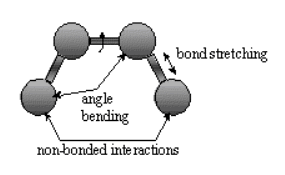Non-bonded atoms (greater than two bonds apart) interact through van der Waals attraction, steric repulsion and electrostatic attraction/repulsion. These properties are easiest to describe mathematically when atoms are considered as spheres of characteristic radii. The object of MM is to predict the energy associated with a given conformation of a molecule. However, MM energies have no meaning as absolute quantities. Only difference in energy between two or more conformations have meaning. A simple MM energy equation is given by: Energy (E) = E Stretch + EBending  + ETorsion  +  E Non-bonded Interactions These equations together with the data (parameters) required to describe the behavior of different kinds of atoms and bonds, is called a force-field. Many different kinds of force-fields have been developed over the years. Some include additional energy terms that describe other kinds of deformations. Some force-fields account for coupling between bending and stretching in adjacent bonds in order to improve the accuracy of the mechanical model.. The mathematical form of the energy terms varies from force-field to force-field. The more common forms will be described. Stretching Energy: Estretch = Sbondskb (r - ro)2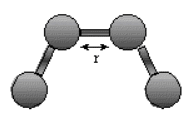The stretching energy equation is based on Hook's law. The kb parameter controls the stiffness of the bond spring, while ro defines its equilibrium length. Unique kb and ro parameters are assigned to each pair of bonded atoms based on their types (e.g. C-C,C-H, etc.). This equation estimates the energy associated with vibration about the equilibrium bond length. This is the equation of a parabola, as can be seen in the following plot: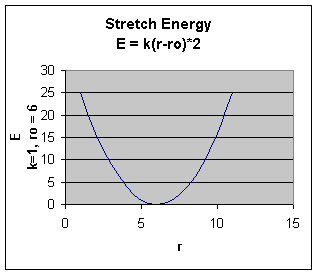Note that the model tends to break down as a bond is stretched toward the point of dissociation. Bending Energy: Ebending = Sangles kQ (Q - Qo)2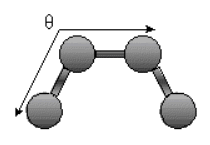. The bending energy equation is also based on Hook's law. The ktheta parameter controls the stiffness of the angle spring, while thetao defines it equilibrium angle. This equation estimates the energy associated with vibration about the equilibrium bond angle.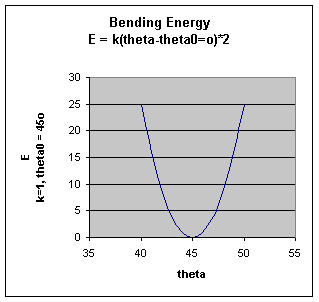Unique parameters for angle bending are assigned to each bonded triplet of atoms based on their types. (e.g. C-C-C, C-O-C, etc.). The effect of the kb and ktheta parameters is to broaden or steepen the slope of the parabola. The larger the value of k, the more energy is required to deform an angle (or bond) from its equilibrium value. Shallow potentials are achieved for k values between 0.0 and 1.0.  Torsion Energy Etorsion = Storsions A [1 + cos ( ntau - phi) ]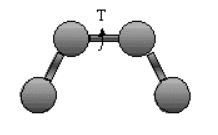The torsion energy is modeled by a s simple periodic function, as can be seen in the following plot: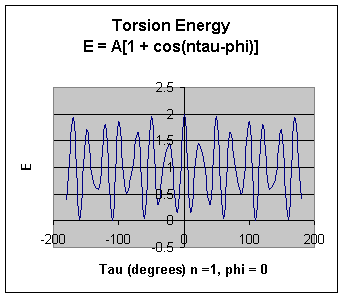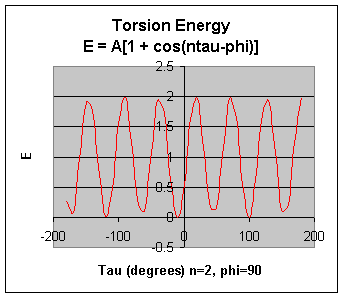The torsion energy in MM is primarily used to correct the remaining energy terms rather than to represent a physical process. The torsional energy represents the amount of energy that must be added to ro subtracted from the Stretching Energy + Bending Energy + Non-Bonded Interaction Energy term to make the total energy agree with experiment or rigorous quantum mechanical calculation for a model dihedral angle (ethane, for example, might be used as a model for an H-C-C-H bond). The A parameter controls the amplitude of the curve, the n parameter controls its periodicity, and phi shifts the entire curve along the rotation angle axis (tau). The parameters are determined from curve fitting. Unique parameters for torsional rotation are assigned to each bonded quartet of atoms based on their types (e.g. C-C-C-C, C-O-C)N, etc.). Torsion potential with 2 combinations of A, n, and phi above. (A=1 in both) Notice that n reflects the type symmetry in the dihedral angle. A CH3-CH3 bond, for example, ought to repeat its energy every 120 degrees. The cis conformation of a dihedral angle is assumed to the zero torsional angle by convention. The parameter phi can be used to synchronize the torsional potential to the initial rotameric state of the molecule whose energy is being computed. Non-Bonded Energy The non-bonded energy represents the pair-wise sum of all the energies of all possible interacting non-bonded atoms I and j: Enonbonding = Si Sj [ -Aij/rij6 + Bij/rij12 ] + Si Sj (qi qj) / rij The first bracketed term above represents van der Waals interactions among the atoms, while the second bracketed term represents Coloumbic electrostatic interactions.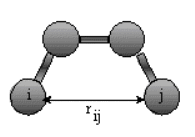The non-bonded energy accounts for repulsion, van der Waals attraction and electrostatic interactions. van der Waals attraction occurs at short range, and rapidly dies off as the interacting atoms move apart by a few angstroms. Repulsion occurs when the distances between interaction atoms becomes even slightly less than the sum of their contact radii. Repulsion is modeled by an equation that is designed to rapidly blow up at close ranges (1/r12 dependency). The energy term that describes attraction/repulsion provides for a smooth transition between the two regimes These effects are often modeling using a 6-12 equation, as show n in the following plot: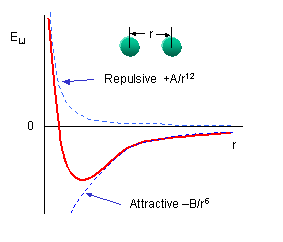I The A and B parameters control the depth and position (interatomic distance) of the potential energy well for a given pair of non-bonded interacting atoms (e.g. C:C, O:C, etc.). In effect, A determined the degree of stickiness of the van der Waals attraction, and B determines the degree of hardness of the atoms (e.g. marshmallow-like, billiard ball-like, etc.). The A parameter can be obtained from atomic polarizability measurements, or it can be calculated quantum mechanically. The B parameter is typically derived from crystallographic data so as to reproduce observed average contact distances between different kinds of atoms in crystals of various molecules.  The equation for the Lennard-Jones potential (often referred to as the 6/12 potential because of the exponents in the equation below)  is: Enonbonding (LJ)  = Si Sj [ -Aij/rij6 + Bij/rij12 ] Lennard-Jones Interactive Applet The electrostatic contribution is modeling using a Coulombic potential The electrostatic energy is a function of the charge on the non-bonded atoms, their interatomic distance, and a molecular dielectric expression that accounts for the attenuation of electrostatic interaction by the environment (e.g. solvent or the molecule itself). Often the molecular dielectric is set to a constant value between 1.0 and 5.0. A linearly varying distance-dependent dielectric (i.e.1.r) is sometimes used to account for the increase in environmental bulk as the separation distance between interacting atoms increases. Partial atomic changes can be calculated for small molecules using an ab initio or semiempirical quantum technique (usually MOPAC or AMPAC).  The equation for the electrostatic potential is: Enonbonding (electrostatic) =  Si Sj (qi qj) / rij   Some programs assign charges using rules or templates, especially for macromolecules. In some force-fields, the torsional potential is calibrated to a particular charge calculation method (rarely made known to the user). Use of a different method can invalidated the force-field consistency.  Sometimes, an additional bonded interaction term, improper dihedrals, are added as illustrated below.  The potential for that is given by the following equation: Eimproper = Sangles kw (w - wo)2   All of the potential energy functions are illustrated in the graph below.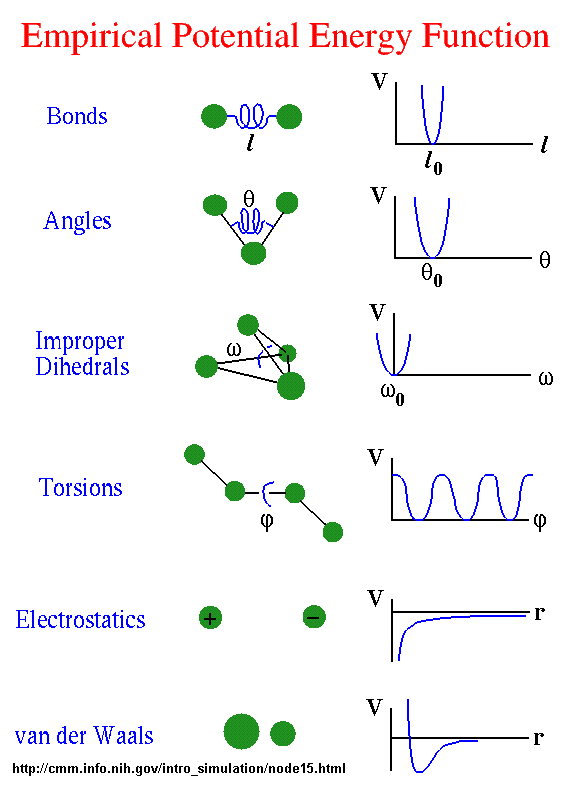Go to this great web site by Sharon Hammes-Schiffer, Shaffer Associate Professor of Chemistry Theoretical and Computational Chemistry at Penn State to see interactive graphs for these potential functions. MOLECULAR DYNAMICS In the broadest sense, MD is concerned with molecular motion . Motion is inherent to all chemical processes. Simple vibrations, like bond stretching, and angle bending, give rise to IR spectra. Chemical reactions, hormone-receptor binding, and other complex processes are associated with many kinds of intra- and intermolecular motions. The driving force for chemical processes is described by thermodynamics. The mechanism by which chemical processes occurs is described by kinetics. Thermodynamics dictates the energetic relationships between different chemical states, whereas the sequence or rate of events that occur as molecules transform between their various possible states is described by kinetics. Conformational transitions and local vibrations are the usual subjects of molecular dynamics studies. MD alters the intramoleuclar degrees of freedom in a step-wise fashion, analogous to energy minimization. The individual steps in energy minimization are merely directed at establishing a down-hill direction to a minimum. The steps in MD, on the other hand, meaningfully represent the changes in atomic position, ri, over time (i.e. velocity). Newton's equation (Fi = mi ai) is used in the MD formalism to simulate atomic motion. The rate and direction of motion (velocity) are governed by the forces that the atoms of the system exert on each other as described by Newton's equation. In practice, the atoms are assigned initial velocities that conform to the total kinetic energy of the system, which in turn, is dictated by the desired simulation temperature. This is carried out by slowly heating the system (initially at absolute zero) and then allowing the energy to equilibrate among the constituent atoms. The basic ingredients of MD are the calculation of the force on each atom, and form that information, the position of each atom through a s specified period of time (typically on the order of picoseconds = 10-12 seconds). The force on an atom can be calculated from the change in energy between its current position and its position a small distance away. This can be recognized as the derivative of the energy with respect to the change in the atom's position: -dE/dri = Fi Energies can be calculated using either MM or quantum mechanics methods. MM energies are limited to applications that do not involve drastic changes in electronic structure such as bond making/breaking. Quantum mechanical energies can be used to study dynamic processes involving chemical changes. The latter technique is extremely novel and of limited availability. Knowledge of the atomic forces and masses can then be used to solve for the positions of each atom along a series of extremely small time steps (on the order of femtoseconds). The resulting series of snapshots of structural changes over time is called a trajectory. The use of this method to compute trajectories can be more easily seen when Newton's equation is expressed in the following form -dE/dri = mi d2ri/dt2 In practice, trajectories are not directly obtained from Newton's equation due to lack of an analytical solution. First the atomic accelerations are computed from the forces and masses. The velocites are next calculated from the accelerations based on the following relationship:ai = dvi/dt. Lastly, the positions are calculated from the velocities: vi = dri/dt. A trajectory between two states can be subdivided into a series of sub-states separated by a small time step, delta t (e.g. 1 fs). The initial atomic positions at time t are used to predict the atomic positions at time t = delta t. The positions at t = delta t are used to predict the positions at t = 2delta t, and so on. The leapfrog method is a common numerical approach to calculating trajectories based on Newton's equation. The method derives its name from the fact that the velocity and position information successively alternate at ½ time step intervals. MD has no defined point of termination other than the amount of time that can be practically covered. Unfortunately, the current ps order of magnitude limit is often not long enough to follow many kinds of state to state transformations, such as large conformational transitions in proteins.Biochemistry - Where molecules become life...